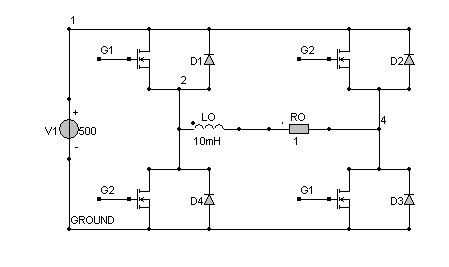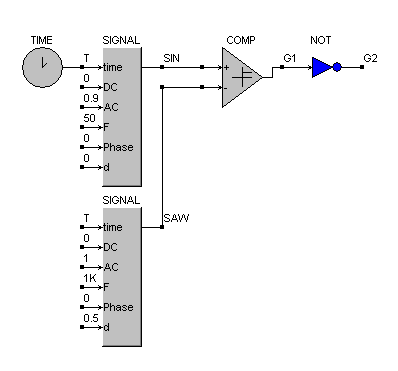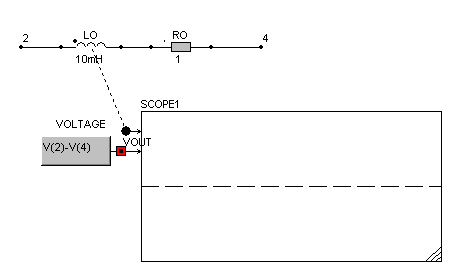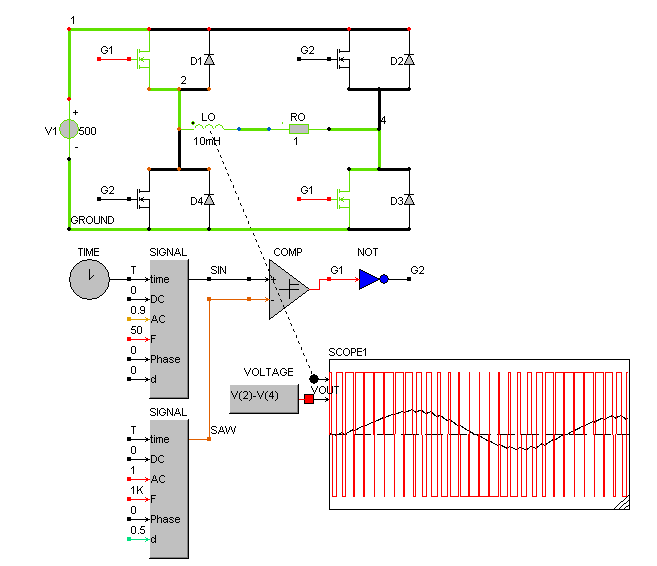• Introduction
• What is in this manual
• What is Caspoc
• User interface
• Introduction
• Starting
• Simulation
• Editing
• Viewing and printing
• Getting Started
• Basic editing
• Simulation in the time domain
• Basic User Interface Topics
• Editing
• Simulation
• Viewing
• Library
• Reports
• Project management
• Circuit and Block Diagram Components
• Introduction
• Cscript and user defined functions
• Component parameters
• Modeling Topics
• Introduction
• Power Electronics
• Semiconductors
• Electrical Machines
• Electrical drives
• Power Systems
• Mechanical Systems
• Thermal Systems
• Magnetic Circuits
• Green Energy
• Coupling to FEM
• Experimenter
• Analog hardware description language
• Embedded C code Export
• Coupling to Spice
• Small Signal Analysis
• Matlab coupling
• Tips and tricks
• Appendices

## PWM Control for a Mosfet in a single phase inverter.

A Pulse Width Modulation (PWM) control is constructed using a comperator and a triangular wave form. We will create a model where the mosfet is controlled from the block diagram where the PWM is modeled. Create an inverter as shown below:The gate of the Mosfet is a block diagram node. This is indicated by the square node. We will control the gate of the upper-left and lower-right Mosfet directly from the comparator output. For the the other two complementary gates the inverted output of the comperator is used. The block SIGNAL is used to create wave forms like square waves, sinusoidal and triangular waveforms. Select the block TIME and SIGNAL from Components/Blocks/Source. The PWM regulator is build from a comparator, a triangular and a reference sinusoidal wave form. The block diagram for the sinusoidal modulation is:Triangular wave form
The block SIGNAL is used for creating the triangular wave form for controlling the gate. Open the block SIGNAL by clicking it with the right mouse button. The third parameter indicates the type of the signal. There are various wave forms possible such as sinusoidal, square wave, triangular, etc. The third parameter is set to 2 to select a triangular wave form. The duty cycle for the triangular wave form, being the last input of the block SIGNAL is set to 0.5. The frequency of the triangular wave form is set to 1kHz with a phase-shift of 0 rad.

Sinusoidal wave form
The block SIGNAL is used for creating the siusoidal wave form being the reference wave form. Open the block SIGNAL by clicking it with the right mouse button. The third parameter indicates the type of the signal. There are various wave forms possible such as sinusoidal, square wave, triangular, etc. The third parameter is set to 3 to select a sinusoidal wave form. The duty cycle for the sinusoidal wave form, being the last input of the block SIGNAL is set to 0 since it is not used.

Time domain
Please note that in most cases the first input has to be connected to the block Time. The label for the output from the block TIME is T and is used also for the first input of the sinusoidal block.

Modulation index
The modulation index is dependent on the amplitude of both the triangular and reference wave form. For both SIGNAL blocks, the DC value is set to 0 and the AC value is set to 1 and 0,9 respectively, to model the modulation index. Use a voltage probe VP2 that is selected from Components/Library/Probes to measure the output voltage across the RL load. The labels of the nodes of the load are 2 and 4, if you use the block VOLTAGE as is done in this sample.The simulation shows the voltage across the RL load and the current through the load.